0

# opencv实战---使用TesseractOCR进行文字识别

• TesseractOCR 是一款由HP实验室开发由 Google 维护的开源 OCR（Optical Character Recognition , 光学字符识别）引擎。
• 简单点说，就是用来做字符识别的，可以识别超过100种语言。也可以用来训练其他的语言。
• 听起来不错，但识别的准确率让人恼火。于是，有人训练出了自己的识别库。## 2、软件安装：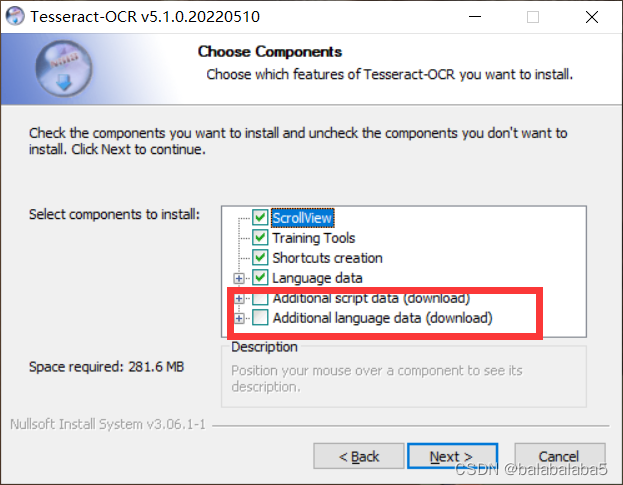• 如果你对自己的梯子速度很有自信的话，请勾选上红色框出来的两项。然后不停的点击next，直到安装成功。
• 如果没有，那咱就不要勾选 。后面也是一路无障碍的安装成功。接下来，咱进入这个github链接，下载所有的文件。下载好压缩包之后，解压，将里面所有的文件都放进刚刚安装tesseract的文件夹里的tessdata里面。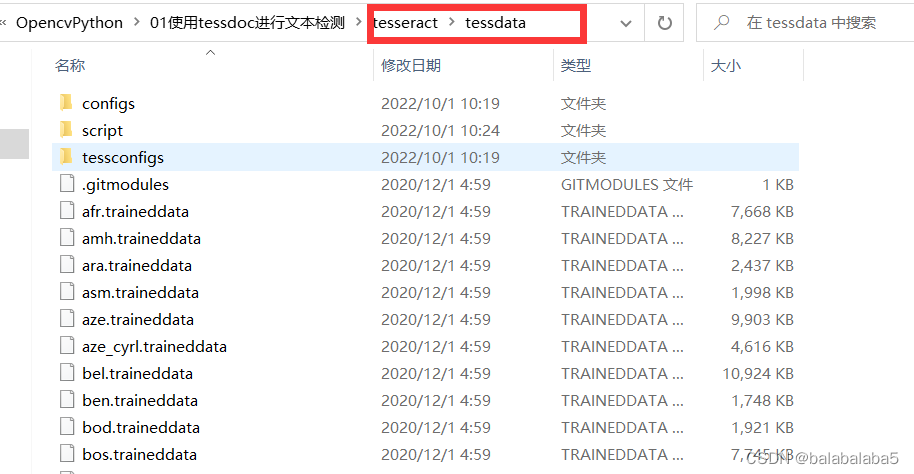## 3、环境变量的设置：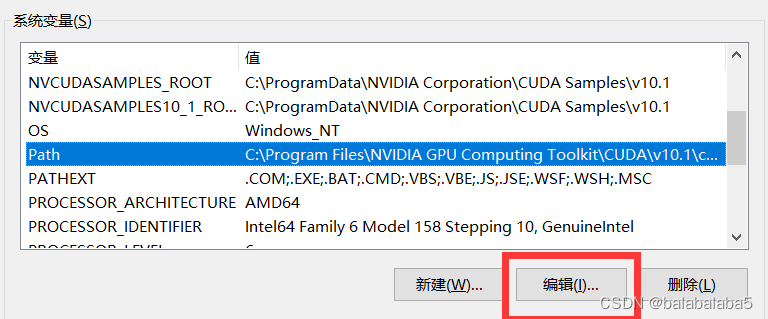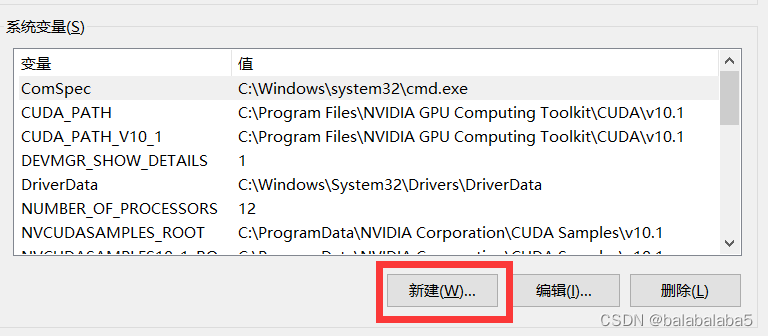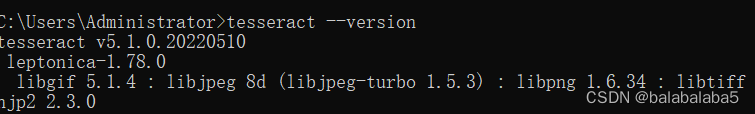## 识别时所需要的库：

``````import cv2
import pytesseract
``````

## 1、先导入咱们安装好的tesseract软件以及所需要识别的图片。

``````pytesseract.pytesseract.tesseract_cmd = 'D:\\OpencvPython\\01使用tessdoc进行文本检测\\tesseract\\tesseract.exe'
img = cv2.cvtColor(img, cv2.COLOR_BGR2RGB)
``````## 2、识别。

``````text = pytesseract.image_to_string(img)
print(text)
``````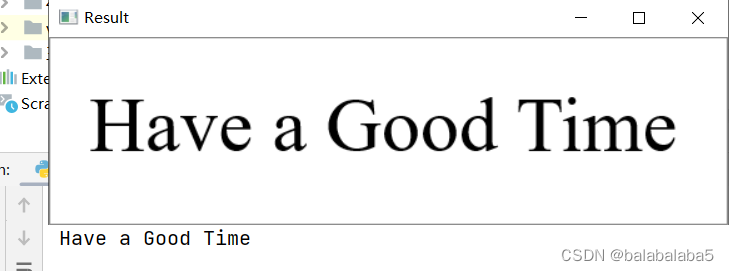## 3、在图片上面框选出字符，并且将字符的识别结果显示在图片上。

``````hImg, wImg, _ = img.shape
boxes = pytesseract.image_to_boxes(img)
for b in boxes.splitlines():
b = b.split(' ')
x1, y1, x2, y2 = int(b), int(b), int(b), int(b)
cv2.rectangle(img, (x1,hImg-y1), (x2, hImg-y2), (0, 0, 255), 2)
cv2.putText(img, b, (x1, hImg-y1+25), cv2.FONT_HERSHEY_SIMPLEX, 1, (50, 50, 255), 2)
``````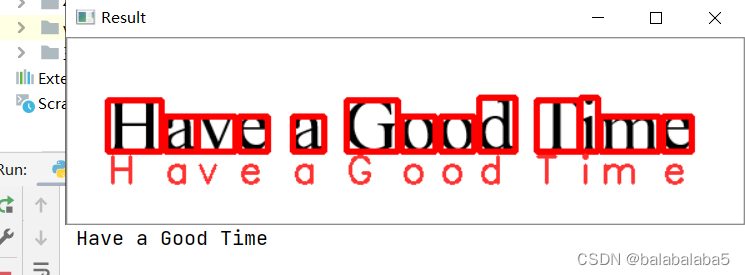hImg, wImg, _ = img.shape
boxes = pytesseract.image_to_boxes(img)

img,shape输出的是原始图片img的高度、宽度、纬度

boxes如下图所示，里面包含着每个字符的识别结果和该字符所在的位置信息。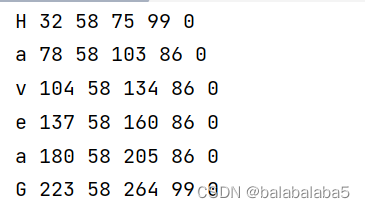for b in boxes.splitlines(): # 这里将boxes的元素一行一行地取出

for b in boxes.splitlines():

``````    b = b.split(' ')           #   这里将b里面的元素，出现空格就分开
``````

b里面的数据：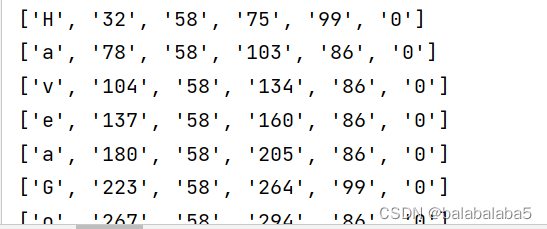hImg : 图片的总高度； wImg : 图片的总宽度

x1: 字符的左下角横坐标； y1：字符的左下角纵坐标

x2: 字符的左上角横坐标； y2：字符的左上角纵坐标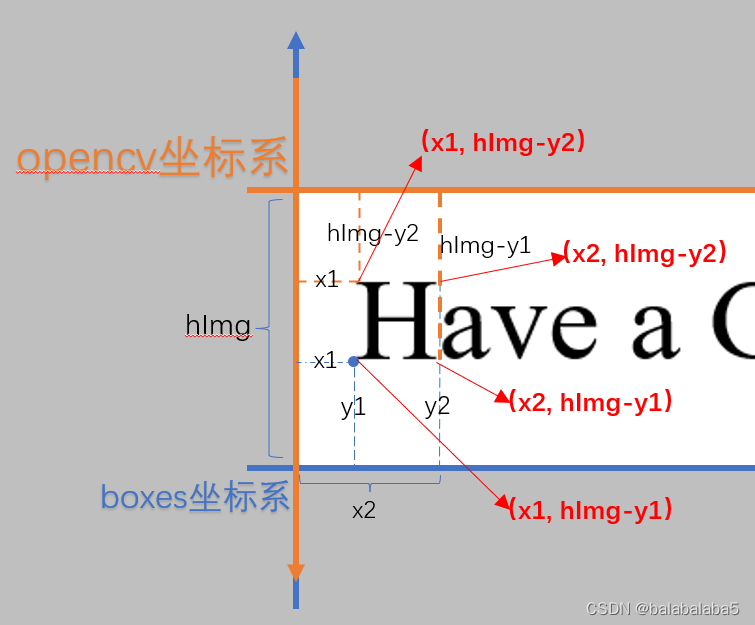• 对于cv2.rectangle函数有个新发现：之前一直以为参数必须是左上角和右下角的坐标确定方框的位置，但现在通过实验验证，是通过确定对角线的方式画出矩形的。无论是主对角线还是副对角线，都可以画出来。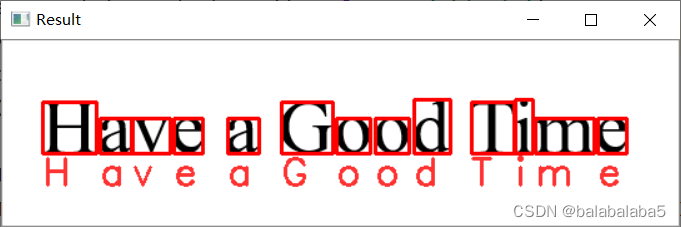## 完整代码：

``````import cv2
import pytesseract

pytesseract.pytesseract.tesseract_cmd = 'D:\\OpencvPython\\01使用tessdoc进行文本检测\\tesseract\\tesseract.exe'
img = cv2.cvtColor(img, cv2.COLOR_BGR2RGB)

text = pytesseract.image_to_string(img)
print(text)

hImg, wImg, _ = img.shape
boxes = pytesseract.image_to_boxes(img)
for b in boxes.splitlines():
b = b.split(' ')
x1, y1, x2, y2 = int(b), int(b), int(b), int(b)
cv2.rectangle(img, (x1,hImg-y1), (x2, hImg-y2), (0, 0, 255), 2)
cv2.putText(img, b, (x1, hImg-y1+25), cv2.FONT_HERSHEY_SIMPLEX, 1, (50, 50, 255), 2)
cv2.imshow('Result', img)
cv2.waitKey(0)
``````

## 英文单词的识别：

``````hImg, wImg, _ = img.shape
boxes = pytesseract.image_to_data(img)
print(boxes)
for x,b in enumerate(boxes.splitlines()):
if x!=0:
b = b.split()
print(b)
if len(b)==12:
x1, y1, x2, y2 = int(b), int(b), int(b), int(b)
cv2.rectangle(img, (x1,y1), (x1+x2, y1+y2), (0, 0, 255), 3)
cv2.putText(img, b, (x1,y1), cv2.FONT_HERSHEY_SIMPLEX, 1, (50, 50, 255), 2)
``````

boxes = pytesseract.image_to_data(img)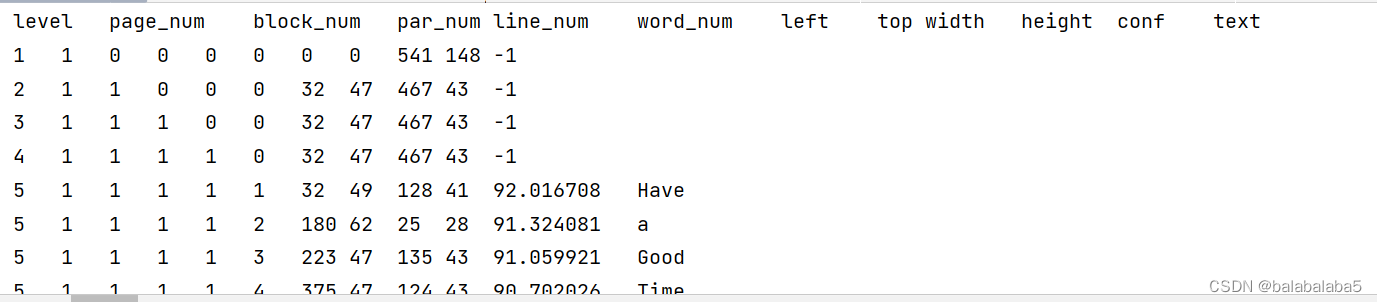for循环的语句里

boxes.splitlines()还是将boxes按行分开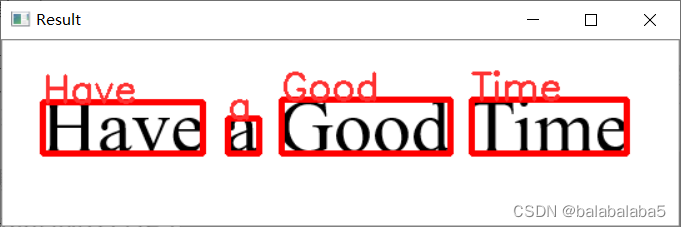## 完整代码：

``````import cv2
import pytesseract

pytesseract.pytesseract.tesseract_cmd = 'D:\\OpencvPython\\01使用tessdoc进行文本检测\\tesseract\\tesseract.exe'
img = cv2.cvtColor(img, cv2.COLOR_BGR2RGB)

text = pytesseract.image_to_string(img)
print(text)

hImg, wImg, _ = img.shape
boxes = pytesseract.image_to_data(img)
print(boxes)
for x,b in enumerate(boxes.splitlines()):
if x!=0:
b = b.split()
print(b)
if len(b)==12:
x1, y1, x2, y2 = int(b), int(b), int(b), int(b)
cv2.rectangle(img, (x1,y1), (x1+x2, y1+y2), (0, 0, 255), 3)
cv2.putText(img, b, (x1,y1), cv2.FONT_HERSHEY_SIMPLEX, 1, (50, 50, 255), 2)
cv2.imshow('Result', img)
cv2.waitKey(0)
``````

## 数字的识别：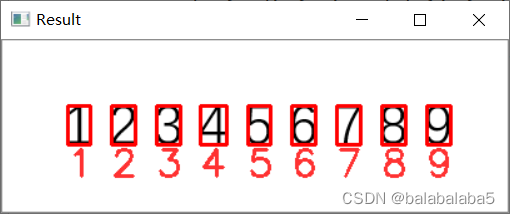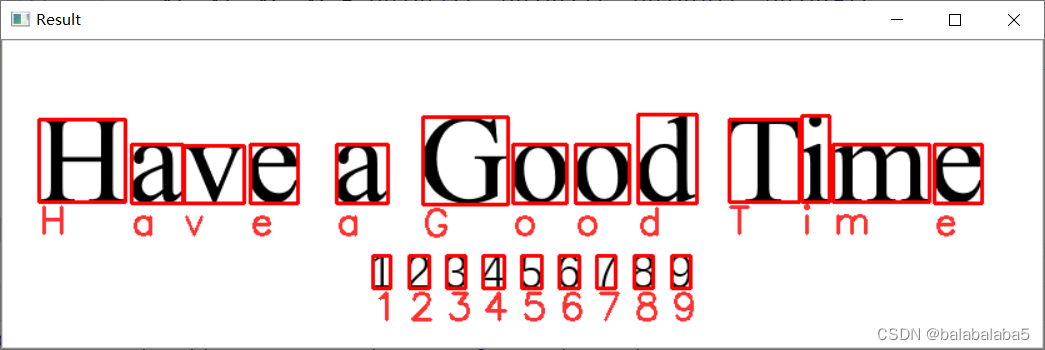cong = r'--oem 3 --psm 6 outputbase digits'

boxes = pytesseract.image_to_boxes(img, config=cong)

-oem 参数控制OCR的引擎模式，控制由超正方体使用的算法类型。

oem的数值代表的模式：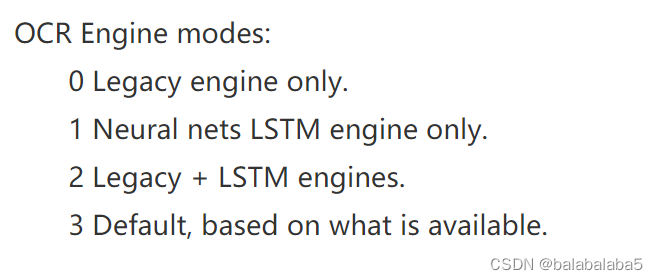-psm 参数控制tesseract使用的自动页面分割模式。

psm的数值代表的模式：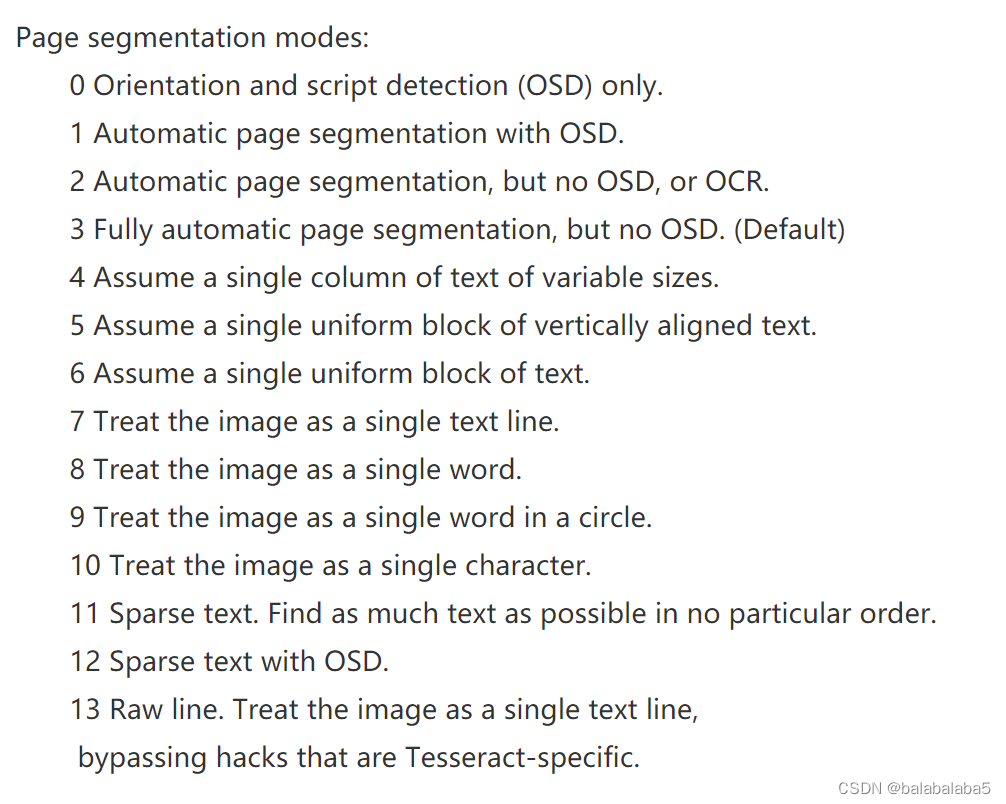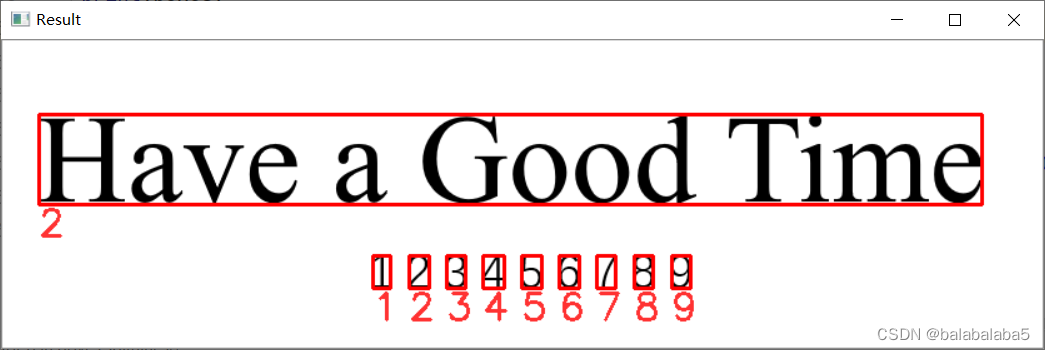## 完整代码：

``````import cv2
import pytesseract

pytesseract.pytesseract.tesseract_cmd = 'D:\\OpencvPython\\01使用tessdoc进行文本检测\\tesseract\\tesseract.exe'
img = cv2.cvtColor(img, cv2.COLOR_BGR2RGB)

text = pytesseract.image_to_string(img)
print(text)

hImg, wImg, _ = img.shape
cong = r'--oem 3 --psm 6 outputbase digits'
boxes = pytesseract.image_to_boxes(img, config=cong)
for b in boxes.splitlines():
b = b.split(' ')
x1, y1, x2, y2 = int(b), int(b), int(b), int(b)
cv2.rectangle(img, (x1,hImg-y1), (x2, hImg-y2), (0, 0, 255), 2)
cv2.putText(img, b, (x1, hImg-y1+25), cv2.FONT_HERSHEY_SIMPLEX, 1, (50, 50, 255), 2)
cv2.imshow('Result', img)
cv2.waitKey(0)
``````

### “opencv实战---使用TesseractOCR进行文字识别”的评论:

##### 关于作者##### overfit同步小助手